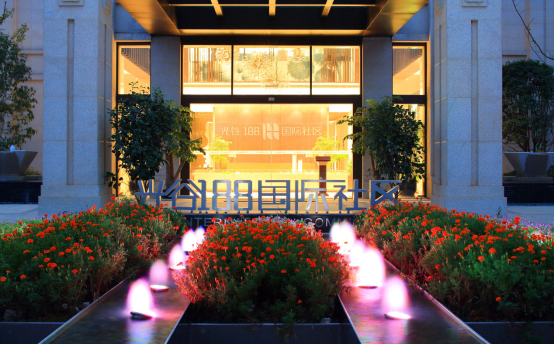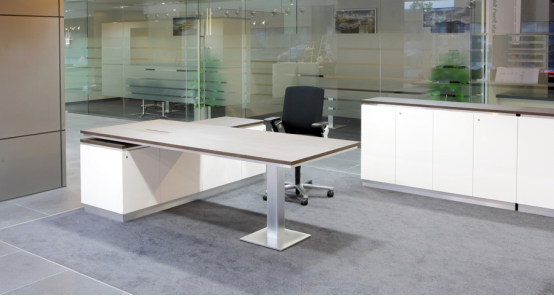|

# 武汉JLAU-Team15年坚持造就室内设计领军

日前，一场盛大的启幕仪式在武汉成功举办，梦幻而又宏大的水上创意T台秀，以及众多房产界、设计圈代表和大咖的捧场，均彰显出这个即将启幕的项目，在武汉房产行业以及设计领域中的分量。这个隆重启幕的项目，正是由郝健沛所带领的JLA,U-Team团队全盘操刀的光谷188国际社区星级会所，整体设计面积超过了6000平米，历时3年完成。然而有趣的是，郝健沛这位项目的“首要功臣”，却并未出现在启幕仪式中，依旧秉持他一贯低调的作风。翻开JLA,U-Team的履历你会发现，除了光谷188国际社区会所之外，包括保时捷亚洲（上海）旗舰店、武汉市土地利用和城市空间规划研究中心（简称“地空中心”）等项目均出自其手，这支由郝健沛历时15年锻造出来的室内设计团队，通过一个个经典案例的完美打造，已经成长为武汉室内设计领域的“王者之师”，下面，我们就来逐层揭开他们的面纱。

最具匠心的顶级设计“从业者”

了解队伍首先要看队伍的核心人物，从不多的媒体报道中，我们可以发现郝健沛不太喜欢抛头露面，是一个喜欢用作品说话的匠心设计师。2002年来到武汉，至今15个年头，从经营JLA的武汉分公司，到创建武汉U-Team，他为这个中原城市带来的不仅仅是国际领先的设计水准，还有欧洲超一流的家居品质，一手打磨出来的“优合模式”更是成为高端设计的代名词，在武汉经济跃居“万亿俱乐部”的过程中，推动武汉设计水平与国际接轨。

这一切都来自于他对高品质近乎苛刻的追求，不仅在设计方案上，在配套的家具上更是如此。目前，U-Team已经建立起与德国DOBERGO、意大利DIEFFEBI等多个欧洲顶级品牌的代理关系，以最高品质护航“所见即所得”的设计目标。此外，他还要求所有员工签署廉政协议，不允许收受任何的回扣，从而进一步确保了团队能够为客户提供最好的产品。

一系列的举措之下，JLA,U-Team也迅速晋升为高端室内设计项目的首选品牌，一个个经典的项目陆续落地武汉，也让郝健沛成为了当地室内设计领域公认的顶级大师。然而，在一些行业性的活动中却鲜有他的身影，“我就是一个设计从业者，干不了明星的活儿，相比于一些所谓的荣誉与名声，我更喜欢的是做出一个好作品后，给大家带来的那份惊奇和幸福。”郝健沛此前曾表示。

坚持国际标准的设计天团

对于国际标准的坚持，是JLA,U-Team团队的一个核心优势，“刚来那几年就像是到非洲去卖鞋，市场都没有形成给设计付费的意识，这一度让我们有些头疼，怀疑自己是不是来早了，但既然来了，降低标准是不可能的，只有输出好的产品，教育市场，相信武汉市场会认可到设计的价值。”郝健沛称。

但正是这份坚持让他牢牢把握住了武汉的市场，客户也从IBM、PHILIPS等国际客户拓展到保利、瑞安、绿地等国内顶级开发商，打造包括武汉瑞安平安大厦、武汉绿地营销中心在内的一系列优秀作品。独创“优合模式”改变行业格局

但仅凭优秀的设计能力在武汉重装修轻设计的早期市场，想要存活下来仍是痴人说梦，这也是一些世界级室内设计公司对武汉市场望而却步的原因。

另一方面，随着武汉经济的发展，室内设计行业也开始“野蛮生长”，比如用套餐式的设计方案去欺骗设计费用，以设计之名去强推家具产品，还有接单后多方吃回扣等现象也屡见不鲜，其所带来的问题不仅仅是花冤枉钱这么简单，更多的是劣质设计所带来的长时间压抑体验。

“优合模式”正是在这样的背景下打磨出来的，JLA团队负责提供设计方案，并从设计的第一视角，甄选最优的家具产品；而U-Team则负责根据方案要求，把欧洲最好品质的材料直接提供给客户，在保证设计主导的同时，最大程度呈现设计效果。

凭借“优合模式”的强力助攻，JLA,U-Team已经稳居武汉室内设计行业第一，并在秉承少即是多的实用设计理念下，让更多好的作品出现在了人们的生活和工作中。“未来，还会尝试将我们的设计能力向其他领域延伸，例如开些设计感强的主题餐厅，将我们的设计能力、家具体系注入线下，把好的设计体验跟美食进行融合，让更多人获得拥有设计的幸福感。”郝健沛说。

`声明：本文由入驻焦点开放平台的作者撰写，除焦点官方账号外，观点仅代表作者本人，不代表焦点立场错误信息举报电话： 400-099-0099，邮箱：jubao@vip.sohu.com，或点此进行意见反馈，或点此进行举报投诉。`A B C D E F G H J K L M N P Q R S T W X Y Z
A - B - C - D - E
• A
• 鞍山
• 安庆
• 安阳
• 安顺
• 安康
• 澳门
• B
• 北京
• 保定
• 包头
• 巴彦淖尔
• 本溪
• 蚌埠
• 亳州
• 滨州
• 北海
• 百色
• 巴中
• 毕节
• 保山
• 宝鸡
• 白银
• 巴州
• C
• 承德
• 沧州
• 长治
• 赤峰
• 朝阳
• 长春
• 常州
• 滁州
• 池州
• 长沙
• 常德
• 郴州
• 潮州
• 崇左
• 重庆
• 成都
• 楚雄
• 昌都
• 慈溪
• 常熟
• D
• 大同
• 大连
• 丹东
• 大庆
• 东营
• 德州
• 东莞
• 德阳
• 达州
• 大理
• 德宏
• 定西
• 儋州
• 东平
• E
• 鄂尔多斯
• 鄂州
• 恩施
F - G - H - I - J
• F
• 抚顺
• 阜新
• 阜阳
• 福州
• 抚州
• 佛山
• 防城港
• G
• 赣州
• 广州
• 桂林
• 贵港
• 广元
• 广安
• 贵阳
• 固原
• H
• 邯郸
• 衡水
• 呼和浩特
• 呼伦贝尔
• 葫芦岛
• 哈尔滨
• 黑河
• 淮安
• 杭州
• 湖州
• 合肥
• 淮南
• 淮北
• 黄山
• 菏泽
• 鹤壁
• 黄石
• 黄冈
• 衡阳
• 怀化
• 惠州
• 河源
• 贺州
• 河池
• 海口
• 红河
• 汉中
• 海东
• 怀来
• I
• J
• 晋中
• 锦州
• 吉林
• 鸡西
• 佳木斯
• 嘉兴
• 金华
• 景德镇
• 九江
• 吉安
• 济南
• 济宁
• 焦作
• 荆门
• 荆州
• 江门
• 揭阳
• 金昌
• 酒泉
• 嘉峪关
K - L - M - N - P
• K
• 开封
• 昆明
• 昆山
• L
• 廊坊
• 临汾
• 辽阳
• 连云港
• 丽水
• 六安
• 龙岩
• 莱芜
• 临沂
• 聊城
• 洛阳
• 漯河
• 娄底
• 柳州
• 来宾
• 泸州
• 乐山
• 六盘水
• 丽江
• 临沧
• 拉萨
• 林芝
• 兰州
• 陇南
• M
• 牡丹江
• 马鞍山
• 茂名
• 梅州
• 绵阳
• 眉山
• N
• 南京
• 南通
• 宁波
• 南平
• 宁德
• 南昌
• 南阳
• 南宁
• 内江
• 南充
• P
• 盘锦
• 莆田
• 平顶山
• 濮阳
• 攀枝花
• 普洱
• 平凉
Q - R - S - T - W
• Q
• 秦皇岛
• 齐齐哈尔
• 衢州
• 泉州
• 青岛
• 清远
• 钦州
• 黔南
• 曲靖
• 庆阳
• R
• 日照
• 日喀则
• S
• 石家庄
• 沈阳
• 双鸭山
• 绥化
• 上海
• 苏州
• 宿迁
• 绍兴
• 宿州
• 三明
• 上饶
• 三门峡
• 商丘
• 十堰
• 随州
• 邵阳
• 韶关
• 深圳
• 汕头
• 汕尾
• 三亚
• 三沙
• 遂宁
• 山南
• 商洛
• 石嘴山
• T
• 天津
• 唐山
• 太原
• 通辽
• 铁岭
• 泰州
• 台州
• 铜陵
• 泰安
• 铜仁
• 铜川
• 天水
• 天门
• W
• 乌海
• 乌兰察布
• 无锡
• 温州
• 芜湖
• 潍坊
• 威海
• 武汉
• 梧州
• 渭南
• 武威
• 吴忠
• 乌鲁木齐
X - Y - Z
• X
• 邢台
• 徐州
• 宣城
• 厦门
• 新乡
• 许昌
• 信阳
• 襄阳
• 孝感
• 咸宁
• 湘潭
• 湘西
• 西双版纳
• 西安
• 咸阳
• 西宁
• 仙桃
• 西昌
• Y
• 运城
• 营口
• 盐城
• 扬州
• 鹰潭
• 宜春
• 烟台
• 宜昌
• 岳阳
• 益阳
• 永州
• 阳江
• 云浮
• 玉林
• 宜宾
• 雅安
• 玉溪
• 延安
• 榆林
• 银川
• Z
• 张家口
• 镇江
• 舟山
• 漳州
• 淄博
• 枣庄
• 郑州
• 周口
• 驻马店
• 株洲
• 张家界
• 珠海
• 湛江
• 肇庆
• 中山
• 自贡
• 资阳
• 遵义
• 昭通
• 张掖
• 中卫

1室1厅1厨1卫1阳台

1
2
3
4
5

0
1
2

1

1

0
1
2
3报名成功，资料已提交审核A B C D E F G H J K L M N P Q R S T W X Y Z
A - B - C - D - E
• A
• 鞍山
• 安庆
• 安阳
• 安顺
• 安康
• 澳门
• B
• 北京
• 保定
• 包头
• 巴彦淖尔
• 本溪
• 蚌埠
• 亳州
• 滨州
• 北海
• 百色
• 巴中
• 毕节
• 保山
• 宝鸡
• 白银
• 巴州
• C
• 承德
• 沧州
• 长治
• 赤峰
• 朝阳
• 长春
• 常州
• 滁州
• 池州
• 长沙
• 常德
• 郴州
• 潮州
• 崇左
• 重庆
• 成都
• 楚雄
• 昌都
• 慈溪
• 常熟
• D
• 大同
• 大连
• 丹东
• 大庆
• 东营
• 德州
• 东莞
• 德阳
• 达州
• 大理
• 德宏
• 定西
• 儋州
• 东平
• E
• 鄂尔多斯
• 鄂州
• 恩施
F - G - H - I - J
• F
• 抚顺
• 阜新
• 阜阳
• 福州
• 抚州
• 佛山
• 防城港
• G
• 赣州
• 广州
• 桂林
• 贵港
• 广元
• 广安
• 贵阳
• 固原
• H
• 邯郸
• 衡水
• 呼和浩特
• 呼伦贝尔
• 葫芦岛
• 哈尔滨
• 黑河
• 淮安
• 杭州
• 湖州
• 合肥
• 淮南
• 淮北
• 黄山
• 菏泽
• 鹤壁
• 黄石
• 黄冈
• 衡阳
• 怀化
• 惠州
• 河源
• 贺州
• 河池
• 海口
• 红河
• 汉中
• 海东
• 怀来
• I
• J
• 晋中
• 锦州
• 吉林
• 鸡西
• 佳木斯
• 嘉兴
• 金华
• 景德镇
• 九江
• 吉安
• 济南
• 济宁
• 焦作
• 荆门
• 荆州
• 江门
• 揭阳
• 金昌
• 酒泉
• 嘉峪关
K - L - M - N - P
• K
• 开封
• 昆明
• 昆山
• L
• 廊坊
• 临汾
• 辽阳
• 连云港
• 丽水
• 六安
• 龙岩
• 莱芜
• 临沂
• 聊城
• 洛阳
• 漯河
• 娄底
• 柳州
• 来宾
• 泸州
• 乐山
• 六盘水
• 丽江
• 临沧
• 拉萨
• 林芝
• 兰州
• 陇南
• M
• 牡丹江
• 马鞍山
• 茂名
• 梅州
• 绵阳
• 眉山
• N
• 南京
• 南通
• 宁波
• 南平
• 宁德
• 南昌
• 南阳
• 南宁
• 内江
• 南充
• P
• 盘锦
• 莆田
• 平顶山
• 濮阳
• 攀枝花
• 普洱
• 平凉
Q - R - S - T - W
• Q
• 秦皇岛
• 齐齐哈尔
• 衢州
• 泉州
• 青岛
• 清远
• 钦州
• 黔南
• 曲靖
• 庆阳
• R
• 日照
• 日喀则
• S
• 石家庄
• 沈阳
• 双鸭山
• 绥化
• 上海
• 苏州
• 宿迁
• 绍兴
• 宿州
• 三明
• 上饶
• 三门峡
• 商丘
• 十堰
• 随州
• 邵阳
• 韶关
• 深圳
• 汕头
• 汕尾
• 三亚
• 三沙
• 遂宁
• 山南
• 商洛
• 石嘴山
• T
• 天津
• 唐山
• 太原
• 通辽
• 铁岭
• 泰州
• 台州
• 铜陵
• 泰安
• 铜仁
• 铜川
• 天水
• 天门
• W
• 乌海
• 乌兰察布
• 无锡
• 温州
• 芜湖
• 潍坊
• 威海
• 武汉
• 梧州
• 渭南
• 武威
• 吴忠
• 乌鲁木齐
X - Y - Z
• X
• 邢台
• 徐州
• 宣城
• 厦门
• 新乡
• 许昌
• 信阳
• 襄阳
• 孝感
• 咸宁
• 湘潭
• 湘西
• 西双版纳
• 西安
• 咸阳
• 西宁
• 仙桃
• 西昌
• Y
• 运城
• 营口
• 盐城
• 扬州
• 鹰潭
• 宜春
• 烟台
• 宜昌
• 岳阳
• 益阳
• 永州
• 阳江
• 云浮
• 玉林
• 宜宾
• 雅安
• 玉溪
• 延安
• 榆林
• 银川
• Z
• 张家口
• 镇江
• 舟山
• 漳州
• 淄博
• 枣庄
• 郑州
• 周口
• 驻马店
• 株洲
• 张家界
• 珠海
• 湛江
• 肇庆
• 中山
• 自贡
• 资阳
• 遵义
• 昭通
• 张掖
• 中卫• 手机• 分享
• 设计
免费设计
• 计算器
装修计算器
• 入驻
合作入驻
• 联系
联系我们
• 置顶
返回顶部